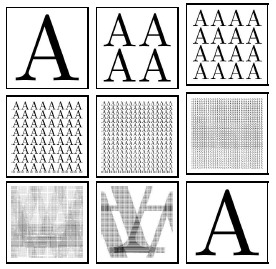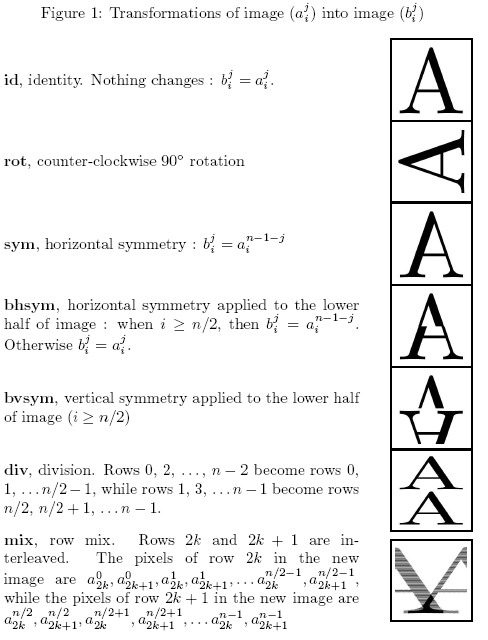Online JudgeProblem SetAuthorsOnline ContestsUser
Web Board
F.A.Qs
Statistical Charts
Problems
Submit Problem
Online Status
Prob.ID:
Register
Authors ranklist
Current Contest
Past Contests
Scheduled Contests
Award Contest
Register
Language:
Pixel Shuffle
 Time Limit: 5000MS Memory Limit: 65536K Total Submissions: 474 Accepted: 193

DescriptionShuffling the pixels in a bitmap image sometimes yields random looking images. However, by repeating the shuffling enough times, one finally recovers the original images. This should be no surprise, since ``shuffling" means applying a one-to-one mapping (or permutation) over the cells of the image, which come in finite number.
Problem
Your program should read a number n , and a series of elementary transformations that define a ``shuffling" φ of n x n images. Then, your program should compute the minimal number m (m > 0) , such that m applications of φ always yield the original n x n image.

For instance if φ is counter-clockwise 90o rotation then m = 4 .Input

Input is made of two lines, the first line is number n ( 2<=n<=210 , n even). The number n is the size of images, one image is represented internally by a n x n pixel matrix (aji) , where i is the row number and j is the column number. The pixel at the upper left corner is at row 0 and column 0.

The second line is a non-empty list of at most 32 words, separated by spaces. Valid words are the keywords id, rot, sym, bhsym, bvsym, div and mix, or a keyword followed by ``-". Each keyword key designates an elementary transform (as defined by Figure 1), and key- designates the inverse of transform key. For instance, rot- is the inverse of counter-clockwise 90o rotation, that is clockwise 90o rotation. Finally, the list k1, k2,..., kp designates the compound transform φ = k1ok2o ... okp . For instance, ``bvsym rot-" is the transform that first performs clockwise 90o rotation and then vertical symmetry on the lower half of the image.Output

Your program should output a single line whose contents is the minimal number m (m > 0) such that φm is the identity. You may assume that, for all test input, you have m < 231 .

Sample Input

```256
rot- div rot div
```

Sample Output

```8
```

Hint

If the input is:
256
bvsym div mix
Then, correct output is:
63457

Source

[Submit]   [Go Back]   [Status]   [Discuss]Home PageGo BackTo top